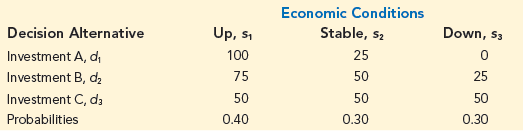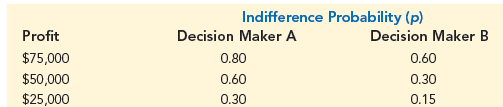A firm has three investment alternatives. Payoffs are in thousands of dollars.a. Using the expected value approach, which decision is preferred?

b. For the lottery having a payoff of \$100,000 with probability p and \$0 with probability (1 – p), two decision makers expressed the following indifference probabilities. Find the most preferred decision for each decision maker using the expected utility approach.c. Why don’t decision makers A and B select the same decision alternative?